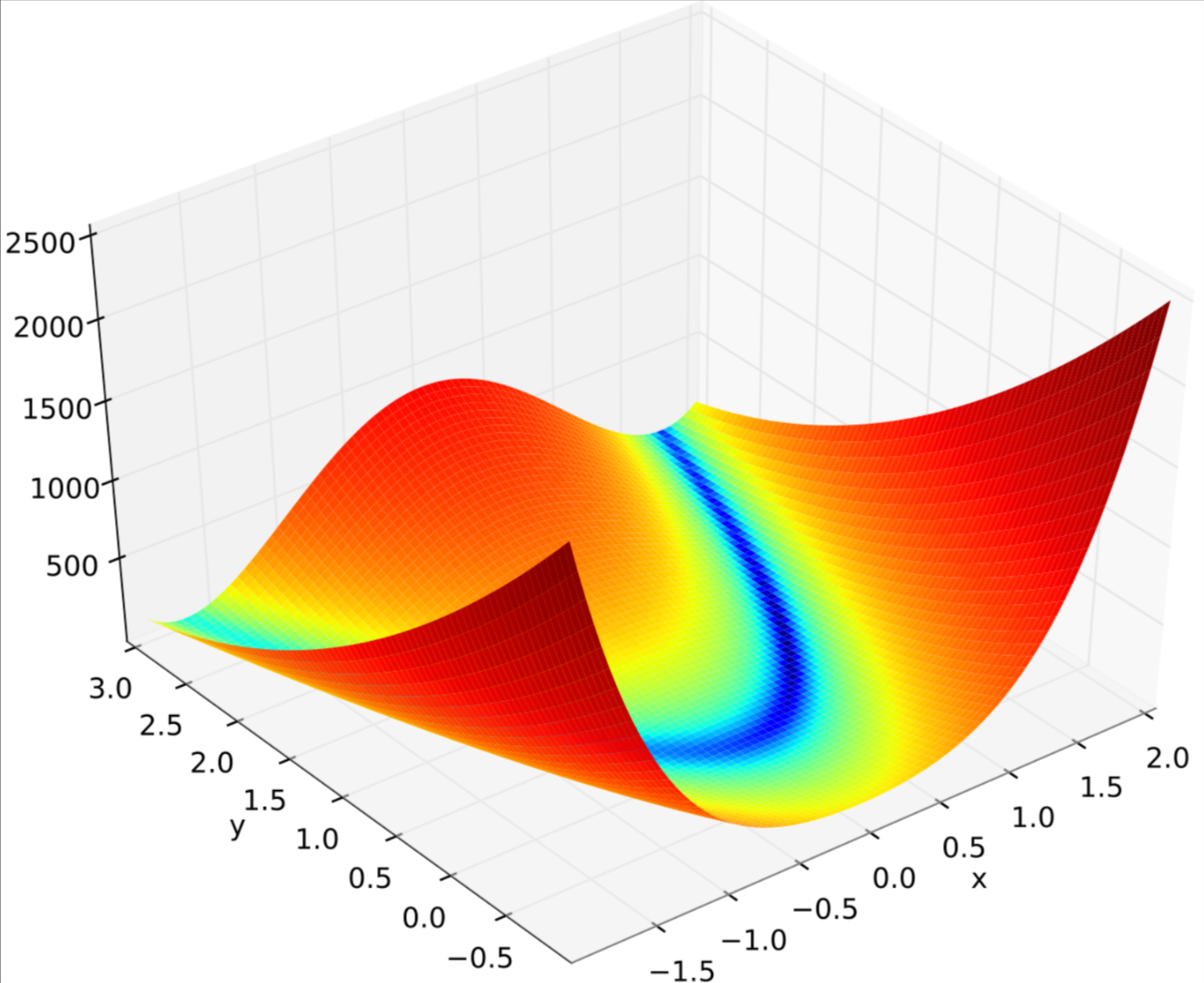﻿ 分别使用 Python 和 Math.Net 调用优化算法_python_开心洋葱网
• 欢迎访问开心洋葱网站，在线教程，推荐使用最新版火狐浏览器和Chrome浏览器访问本网站，欢迎加入开心洋葱` QQ群`
• 为方便开心洋葱网用户，开心洋葱官网已经开启复制功能！
• 欢迎访问开心洋葱网站，手机也能访问哦~欢迎加入开心洋葱多维思维学习平台` QQ群`
• 如果您觉得本站非常有看点，那么赶紧使用Ctrl+D 收藏开心洋葱吧~~~~~~~~~~~~~！
• 由于近期流量激增，小站的ECS没能经的起亲们的访问，本站依然没有盈利，如果各位看如果觉着文字不错，还请看官给小站打个赏~~~~~~~~~~~~~！

# 分别使用 Python 和 Math.Net 调用优化算法

2868次浏览 手机上查看

[隐藏]

## 1.1. Rosenbrock 函数

Rosenbrock 函数的定义如下：

`f(x)=100(y−x2)2+(1−x)2`

Rosenbrock 函数的每个等高线大致呈抛物线形，其全域最小值也位在抛物线形的山谷中（香蕉型山谷）。很容易找到这个山谷，但由于山谷内的值变化不大，要找到全域的最小值相当困难。## 2.2. Python

Python 里面的 scipy.optimize 提供了丰富的优化算法，对于 Rosenbrock函数，它的求解代码如下：

``````import numpy as np
from scipy.optimize import minimize
def rosenbrock(x):
return (1 - x)**2 + 100 * ((x - x * x)**2)
x0 = np.array([1.2, 1.2])
best = minimize(rosenbrock, x0)
print(best)
``````

`minimize` 有两个参数，其中 `rosenbrock` 是要去求得最小值得 objective function；`x0` 是初始值，有时候初始值对结果影响很大。

``````     fun: 3.3496916936926394e-12
hess_inv: array([[0.49944334, 0.99865554],
[0.99865554, 2.00167338]])
jac: array([-4.95083209e-05,  2.79682766e-05])
message: 'Desired error not necessarily achieved due to precision loss.'
nfev: 159
nit: 10
njev: 49
status: 2
success: False
x: array([0.99999874, 0.9999976 ])
``````

``````final_simplex: (array([[0.999993  , 0.99998474],
[0.99995096, 0.99990431],
[1.00003347, 1.00007239]]), array([2.05633807e-10, 2.97215547e-09, 4.09754011e-09]))
fun: 2.0563380675204333e-10
message: 'Optimization terminated successfully.'
nfev: 82
nit: 43
status: 0
success: True
x: array([0.999993  , 0.99998474])
``````

## 3.3. Math.Net

Math.Net 是一个开源项目，旨在构建和维护涵盖基础数学的工具箱，以满足 .Net 开发人员的高级需求和日常需求。其中 Math.NET Numerics 旨在为科学、工程和日常使用中的数值计算提供方法和算法。涵盖的主题包括特殊函数，线性代数，概率模型，随机数，插值，积分变换等等。

``````Install-Package MathNet.Numerics
``````

``````using MathNet.Numerics.LinearAlgebra;
using MathNet.Numerics.LinearAlgebra.Double;
using MathNet.Numerics.Optimization;
using System;

double Value(Vector<double> input)
{
return Math.Pow((1 - input), 2) + 100 * Math.Pow((input - input * input), 2);
}
var obj = ObjectiveFunction.Value(Value);
var solver = new NelderMeadSimplex(convergenceTolerance: 0.0000000001, maximumIterations: 1000);
var initialGuess = new DenseVector(new[] { 1.2, 1.2 });

var result = solver.FindMinimum(obj, initialGuess);
Console.WriteLine("Value:\t" + result.FunctionInfoAtMinimum.Value);
Console.WriteLine("Point:\t" + result.MinimizingPoint + " , " + result.MinimizingPoint);
Console.WriteLine("Iterations:\t" + result.Iterations);
``````

``````Value:  5.352382362443507E-19
Point:  1.0000000007114838 , 1.0000000014059296
Iterations:     145
``````

## 4.4. 最后

Math.Net 提供了很多多元函数局部最小值的算法，但比起 Python 还是简化了太多，例如我还搞不清楚 Math.Net 中的优化算法怎么添加约束条件，这方面有机会再研究研究。

[开心洋葱]• 版权声明

本站的文章和资源来自互联网或者站长的原创，按照 CC BY -NC -SA 3.0 CN协议发布和共享，转载或引用本站文章应遵循相同协议。如果有侵犯版权的资源请尽快联系站长，我们会在24h内删除有争议的资源。
• 合作网站

• 友情链接

• 关于我们

一群热爱思考，热爱生活，有理想的新社会主义接班人的多维思维学习平台，天行健，君子以自强不息。地势坤，君子以厚德载物。
……